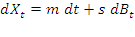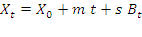# Latest content was relocated to https://bintanvictor.wordpress.com. This old blog will be shutdown soon.

## Tuesday, March 17, 2015

### using dB_t vs B_t in a formula

label - stoch

(dW is a common synonym of dB)

Whenever I see dB, I used to see it as a differential of "B". But it's undefined – no such thing as a differential for a Brownian motion!

Actually any dB equation is an integral equation involving a "stochastic integral" which has a non-trivial definition. Greg Lawler spent a lot of time explaining the meaning of a stochastic integral.

I seldom see B itself, rather than dB, used in a formula describing a random process. The one big exception is the exact formula describing a GBM process.Greg confirmed that the regular BM can also be described in B rather than dB:

givenThis signal-noise formula (see other post) basically says random walk position X at time t is a non-random, predictable position with an chance element superimposed. The chance element is a random variable ~ N(mean 0, variance s2t).

This is actually the most precise description of the random process X(t).

We can also see this as a generalized Brownian motion, expressed using a Standard Brownian motion. Specifically, it adds drift rate and variance parameter to the SBM.